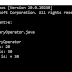are special symbols that perform specific operations on one, two, or three operands, and then return a result. There are different types of operators are available in java which are given below:
1. Arithmetic Operators.
2. Unary Operators.
3. Assignment Operators.
4. Relational Operators.
5. Logical Operators.
6. BitWise Operators.
7. Ternary Operators.
8. InstanceOf Operators.

## Java Ternary Operator:

Ternary Operator in java is also known as the conditional operator. This operator takes three operands. This operator can replace certain types of if-then-else statements. The syntax of the ternary operator is shown below:

`` variable = expression1 ? expression2: expression3 ``

Here, If expression1  is true, then expression2 is evaluated; otherwise, expression3 is evaluated.

## Java Ternary Example to find the largest among two numbers:

``````class TernaryOperator{
public static void main(String[] args)
{

// variable declaration
int num1 = 20, num2 = 30, max;

System.out.println("First number is: " + num1);
System.out.println("Second number is: " + num2);

// Largest among num1 and num2
max = (num1 > num2) ? num1 : num2;

// Print the largest number
System.out.println("Maximum Number is: = " + max);
}
}
```
```

Output:

You may also like these related posts:

1. Bitwise operator in java with example.

Java Ternary Operator with Example | Java Conditional OperatorReviewed by Prashant Srivastava on December 15, 2019 Rating: 5